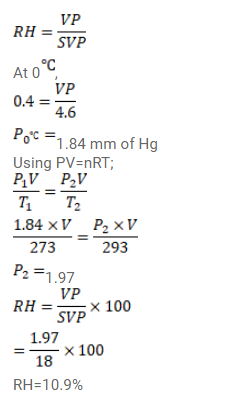# Use R=8.3 J/mol-K wherever required. On a winter day, the outside temperature is 0°CQuestion:

Use $R=8.3 \mathrm{~J} / \mathrm{mol}-\mathrm{K}$ wherever required.

On a winter day, the outside temperature is $0^{\circ} \mathrm{C}$ and relative humidity $40 \%$. The air from outside comes into a room and is heated to $20^{\circ} \mathrm{C}$. What is the relative humidity in the room? The saturation vapour pressure at $0^{\circ} \mathrm{C}$ is $4.6 \mathrm{~mm}$ of mercury and at $20^{\circ} \mathrm{C}$ it is $18 \mathrm{~mm}$ of mercury.

Solution: